ANOVA or linear mixed model?

HomeBlogs# ANOVA or linear mixed model?

The VSNi Team

13 April 2022Analysis of variance (ANOVA) is a widely used statistical technique for analysing data from designed experiments. However, over recent decades, many analysts are now using linear mixed models (LMMs), also known as multi-level models, to analyse data from designed experiments instead of ANOVA. So, which method should you use?

Like ANOVA, LMMs can analyse data with more than one source of variation, in addition to the usual residual term (e.g., data from a split-plot experiment with whole-plot and sub-plot error terms). And for balanced data sets, the LMM results are the same as those from ANOVA. However, there are several important advantages of LMMs over ANOVA. LMMs can:

* Analyse unbalanced data sets (e.g., unbalanced designs or data sets containing missing values).

* Model correlations between observations (e.g., repeated measures data or spatial data).

* Allow for unequal variances between groups.

Genstat has a very powerful set of ANOVA and LMM tools that are straightforward and easy to use. In Genstat, the REML algorithm is used to fit LMMs.

So why use ANOVA at all? For balanced data sets, although the LMM and ANOVA results are the same, LMM cannot provide an analysis-of-variance table. Also, the ANOVA algorithm is much more computationally efficient than the REML algorithm for fitting LMMs, so it is better to use ANOVA whenever possible.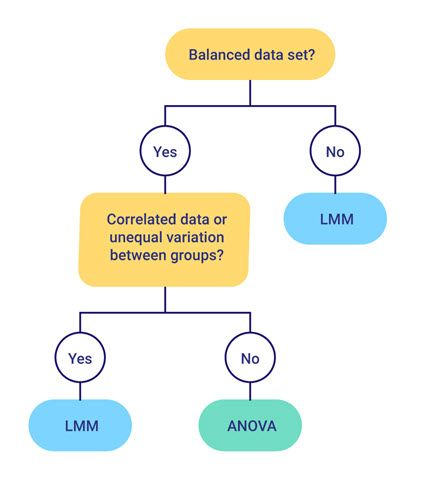#### Comparison of ANOVA and LMM analyses using a real-world example balanced data set

An experiment was conducted to study the effect of two meat-tenderizing chemicals and three temperatures on the force required to break strips of meat. The two hind legs were taken from four carcasses of beef and one leg was treated with chemical 1 and the other with chemical 2. Three sections were then cut from each leg and randomly allocated to three cooking temperatures. All 24 strips of meat (4 carcasses × 2 legs × 3 sections) were cooked in separate ovens.

Notice that this experiment has a hierarchical “split-plot” design, with sections (i.e., sub-plots) nested with legs (i.e., whole-plots) nested with carcasses (i.e., blocks). The two chemicals were randomly allocated to the two legs within each carcass, and the three temperatures to the three sections within each leg.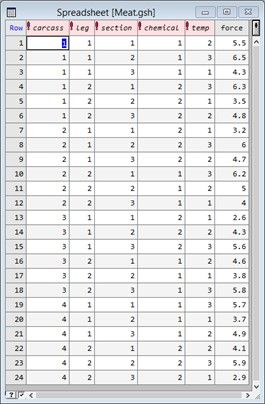Let’s analyse the experimental data using both ANOVA and a LMM, and compare the results.

To analyse the data in Genstat using ANOVA, from the menu select Stats|Analysis of Variance|General.

The model is specified by populating the Y-variate, Treatment structure and Block structure fields as shown below.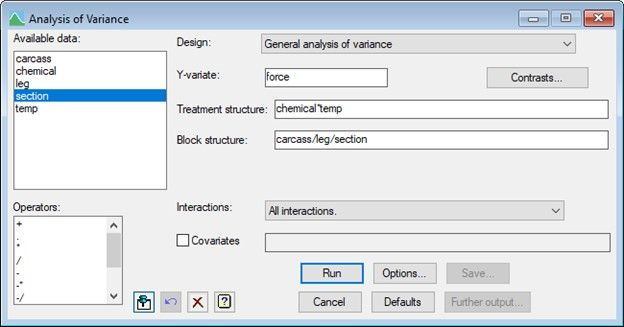The ANOVA table shows that we have three strata (highlighted yellow) in the hierarchy, corresponding to the three random terms: carcass (blocks), leg within the carcass (whole-plots), and section within leg within carcass (sub-plots). The analysis automatically works out for which strata each fixed (or treatment) term is estimated and compares it with the correct residual. So, the sum of squares for the chemical is compared with a residual which represents the random variability of the legs within carcass (A). Conversely, temp and the chemical by temp interaction are compared with the residual for sections within legs (B)

.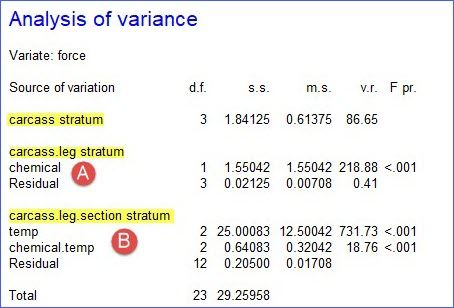To analyse the data using a LMM, from the menu select Stats|Mixed Models (REML)|Linear Mixed Models. The response, fixed terms and random terms in the model are specified as shown below.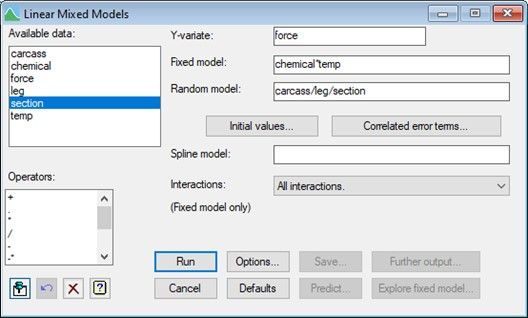Genstat estimates a variance component for every term in the random model (A), apart from the residual. The residual (B) is a random term with a value for every unit in the design, here the 24 (4 carcasses × 2 legs × 3 sections) strips of meat. The variance component of a term measures the inherent variability of that term over and above the variability of the sub-units of which it is composed. Generally, this is positive, indicating that the units become more variable the larger they become. However, in this example the variance component for carcass.leg is negative, indicating that the legs are less variable than the sections within the legs. (This is the same conclusion we draw from the analysis of variance above by comparing the residuals in the different strata.)

The significance of the fixed terms are assessed using F statistics (C), or, if the denominator degrees of freedom can’t be estimated, the less reliable Wald tests are used. For orthogonal (i.e., balanced) designs, the F statistics and p-values will be identical to those generated by ANOVA.  For non-orthogonal designs, the F statistics have approximate F-distributions and the order of the tests is important!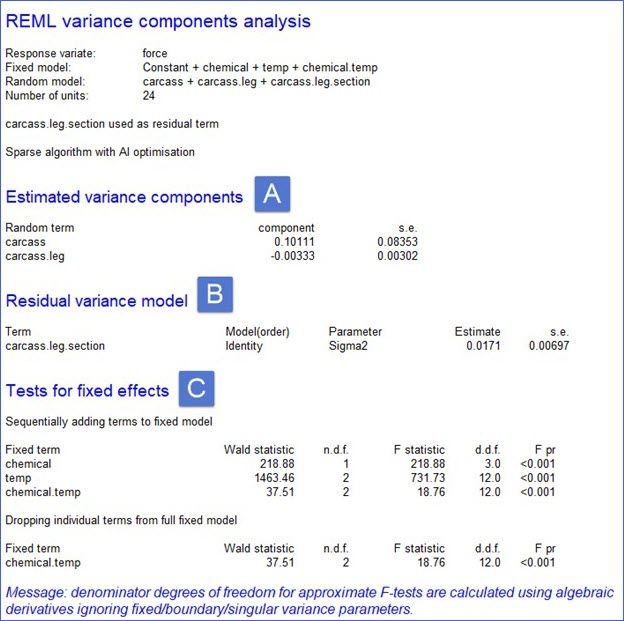As we have seen in the above example, for balanced data LMM and ANOVA give the same result. However, as highlighted earlier, ANOVA is preferred as it provides an analysis of variance table and the ANOVA algorithm is much more efficient than the REML algorithm used to fit the LMM.

Kanchana Punyawaew

01 March 2021

Linear mixed models: a balanced lattice square

This blog illustrates how to analyze data from a field experiment with a balanced lattice square design using linear mixed models. We’ll consider two models: the balanced lattice square model and a spatial model.

The example data are from a field experiment conducted at Slate Hall Farm, UK, in 1976 (Gilmour et al., 1995). The experiment was set up to compare the performance of 25 varieties of barley and was designed as a balanced lattice square with six replicates laid out in a 10 x 15 rectangular grid. Each replicate contained exactly one plot for every variety. The variety grown in each plot, and the coding of the replicates and lattice blocks, is shown in the field layout below: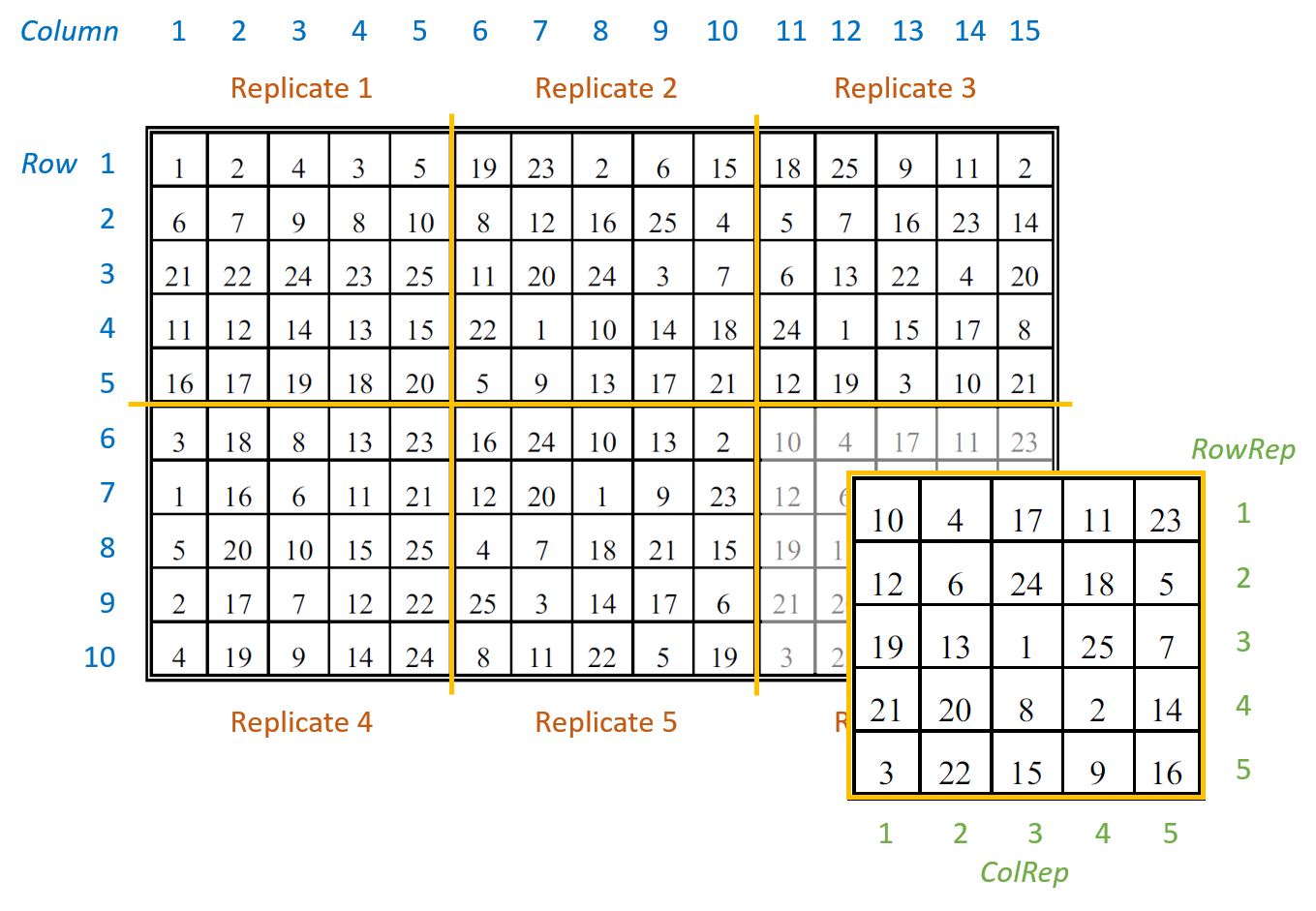There are seven columns in the data frame: five blocking factors (Rep, RowRep, ColRep, Row, Column), one treatment factor, Variety, and the response variate, yield.The six replicates are numbered from 1 to 6 (Rep). The lattice block numbering is coded within replicates. That is, within each replicates the lattice rows (RowRep) and lattice columns (ColRep) are both numbered from 1 to 5. The Row and Column factors define the row and column positions within the field (rather than within each replicate).

### Analysis of a balanced lattice square design

To analyze the response variable, yield, we need to identify the two basic components of the experiment: the treatment structure and the blocking (or design) structure. The treatment structure consists of the set of treatments, or treatment combinations, selected to study or to compare. In our example, there is one treatment factor with 25 levels, Variety (i.e. the 25 different varieties of barley). The blocking structure of replicates (Rep), lattice rows within replicates (Rep:RowRep), and lattice columns within replicates (Rep:ColRep) reflects the balanced lattice square design. In a mixed model analysis, the treatment factors are (usually) fitted as fixed effects and the blocking factors as random.

The balanced lattice square model is fitted in ASReml-R4 using the following code:

> lattice.asr <- asreml(fixed = yield ~ Variety,
random = ~ Rep + Rep:RowRep + Rep:ColRep,
data=data1)

The REML log-likelihood is -707.786.

The model’s BIC is:The estimated variance components are:The table above contains the estimated variance components for all terms in the random model. The variance component measures the inherent variability of the term, over and above the variability of the sub-units of which it is composed. The variance components for Rep, Rep:RowRep and Rep:ColRep are estimated as 4263, 15596, and 14813, respectively. As is typical, the largest unit (replicate) is more variable than its sub-units (lattice rows and columns within replicates). The "units!R" component is the residual variance.

By default, fixed effects in ASReml-R4 are tested using sequential Wald tests: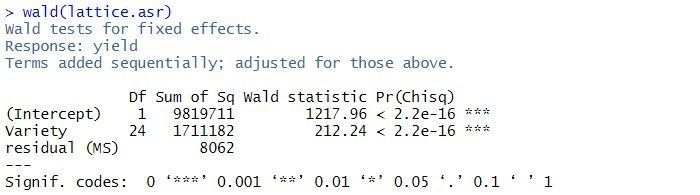In this example, there are two terms in the summary table: the overall mean, (Intercept), and Variety. As the tests are sequential, the effect of the Variety is assessed by calculating the change in sums of squares between the two models (Intercept)+Variety and (Intercept). The p-value (Pr(Chisq)) of  < 2.2 x 10-16 indicates that Variety is a highly significant.

The predicted means for the Variety can be obtained using the predict() function. The standard error of the difference between any pair of variety means is 62. Note: all variety means have the same standard error as the design is balanced.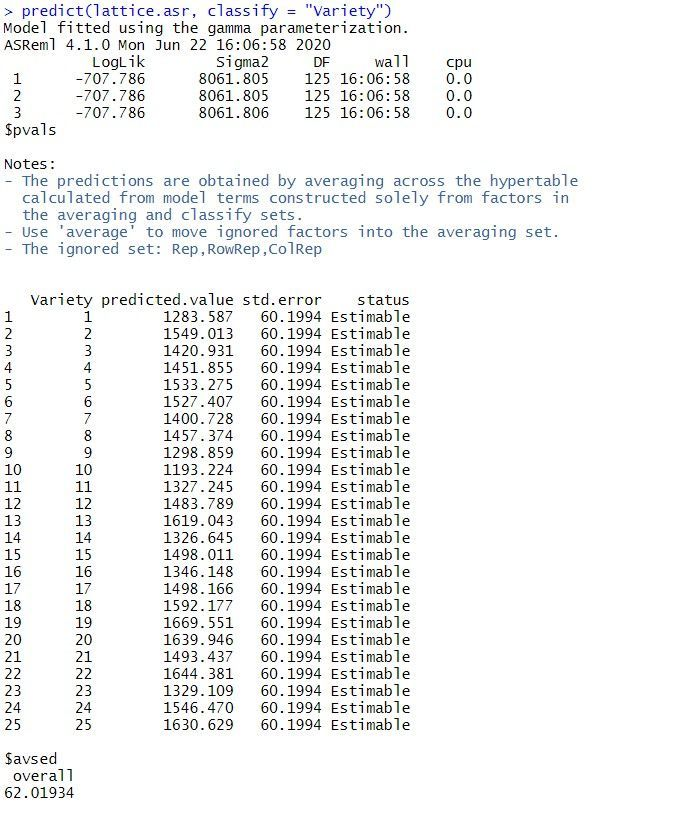Note: the same analysis is obtained when the random model is redefined as replicates (Rep), rows within replicates (Rep:Row) and columns within replicates (Rep:Column).

### Spatial analysis of a field experiment

As the plots are laid out in a grid, the data can also be analyzed using a spatial model. We’ll illustrate spatial analysis by fitting a model with a separable first order autoregressive process in the field row (Row) and field column (Column) directions. This is often a useful model to start the spatial modeling process.

The separable first order autoregressive spatial model is fitted in ASReml-R4 using the following code:

> spatial.asr <- asreml(fixed = yield ~ Variety,
residual = ~ar1(Row):ar1(Column),
data = data1)

The BIC for this spatial model is:The estimated variance components and sequential Wald tests are: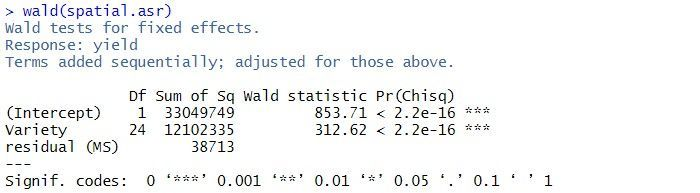The residual variance is 38713, the estimated row correlation is 0.458, and the estimated column correlation is 0.684. As for the balanced lattice square model, there is strong evidence of a Variety effect (p-value < 2.2 x 10-16).

A log-likelihood ratio test cannot be used to compare the balanced lattice square model with the spatial models, as the variance models are not nested. However, the two models can be compared using BIC. As the spatial model has a smaller BIC (1415) than the balanced lattice square model (1435), of the two models explored in this blog, it is chosen as the preferred model. However, selecting the optimal spatial model can be difficult. The current spatial model can be extended by including measurement error (or nugget effect) or revised by selecting a different variance model for the spatial effects.

#### References

Butler, D.G., Cullis, B.R., Gilmour, A. R., Gogel, B.G. and Thompson, R. (2017). ASReml-R Reference Manual Version 4. VSN International Ltd, Hemel Hempstead, HP2 4TP UK.

Gilmour, A.R., Thompson, R. & Cullis, B.R. (1995). Average Information REML, an efficient algorithm for variance parameter estimation in linear mixed models. Biometrics, 51, 1440-1450.

Dr. Vanessa Cave

13 December 2021

ANOVA, LM, LMM, GLM, GLMM, HGLM? Which statistical method should I use?

Unsure which statistical method is appropriate for your data set? Want to know how the different methods relate to each one another?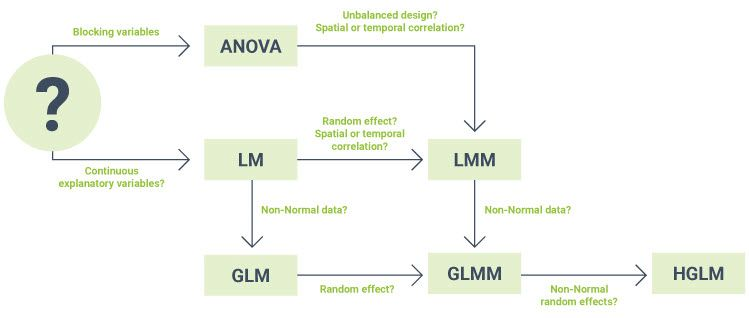Dr Vanessa Cave is an applied statistician interested in the application of statistics to the biosciences, in particular agriculture and ecology, and is a developer of the Genstat statistical software package. She has over 15 years of experience collaborating with scientists, using statistics to solve real-world problems.  Vanessa provides expertise on experiment and survey design, data collection and management, statistical analysis, and the interpretation of statistical findings. Her interests include statistical consultancy, mixed models, multivariate methods, statistical ecology, statistical graphics and data visualisation, and the statistical challenges related to digital agriculture.

Vanessa is currently President of the Australasian Region of the International Biometric Society, past-President of the New Zealand Statistical Association, an Associate Editor for the Agronomy Journal, on the Editorial Board of The New Zealand Veterinary Journal and an honorary academic at the University of Auckland. She has a PhD in statistics from the University of St Andrew.

09 March 2022

Meta analysis using linear mixed models

Meta-analysis is a statistical tool that allows us to combine information from related, but independent studies, that all have as an objective to estimate or compare the same effects from contrasting treatments. Meta-analysis is widely used in many research areas where an extensive literature review is performed to identify studies that had a similar research question. These are later combined using meta-analysis to estimate a single combined effect. Meta-analyses are commonly used to answer healthcare and medical questions, where they are widely accepted, but they also are used in many other scientific fields.

By combining several sources of information, meta-analyses have the advantage of greater statistical power, therefore increasing our chance of detecting a significant difference. They also allow us to assess the variability between studies, and help us to understand potential differences between the outcomes of the original studies.

The underlying premise in meta-analysis is that we are collecting information from a group of, say n, studies that individually estimated a parameter of interest, say . It is reasonable to consider that this parameter has some statistical properties. Mainly we assume that it belongs to a Normal distribution with unknown mean and variance. Hence, mathematically we say:

In meta-analysis, the target population parameter θ can correspond to any of several statistics, such as a treatment mean, a difference between treatments; or more commonly in clinical trials, the log-odds ratio or relative risk.

There are two models that are commonly used to perform meta-analyses: the fixed-effect model and the random-effects model. For the fixed-effect model, it is assumed that there is only a single unique true effect our single θ above, which is estimated from a random sample of studies. That is, the fixed-effect model assumes that there is a single population effect, and the deviations obtained from the different studies are only due to sampling error or random noise. The linear model (LM) used to describe this process can be written as:

where is each individual observed response, is the population parameter (also often known as  μ, the overall mean), and is a random error or residual with assumptions of . The variance component is a measurement of our uncertainty in the information (i.e., response) of each study. The above model can be easily fitted under any typical LM routine, such as R, SAS, GenStat and ASReml.

For the random-effects model we still assume that there is a common true effect between studies, but in addition, we allow this effect to vary between studies. Variation between these effects is a reasonable assumption as no two studies are identical, differing in many aspects; for example, different demographics in the data, slightly differing measurement protocols, etc. Because, we have a random sample of studies, then we have a random sample of effects, and therefore, we define a linear mixed model (LMM) using the following expression:

where, as before, is each individual observed response, is the study-specific population parameter, with the assumption of and is a random error or residual with the same normality assumptions as before. Alternatively, the above LMM can be written as:

where and is a random deviation from the overall effect mean θ with assumptions .

This is a LMM because we have, besides the residual, an additional random component that has a variance component associated to it, that is or . This variance is a measurement of the variability ‘between’ studies, and it will reflect the level of uncertainty of observing a specific  . These LMMs can be fitted, and variance components estimated, under many linear mixed model routines, such as nlme in R, proc mixed in SAS, Genstat or ASReml.

Both fixed-effect and random-effects models are often estimated using summary information, instead of the raw data collected from the original study. This summary information corresponds to estimated mean effects together with their variances (or standard deviations) and the number of samples or experimental units considered per treatment. Since the different studies provide different amounts of information, weights should be used when fitting LM or LMM to summary information in a meta-analysis, similar to weighted linear regression. In meta-analysis, each study has a different level of importance, due to, for example, differing number of experimental units, slightly different methodologies, or different underlying variability due to inherent differences between the studies. The use of weights allows us to control the influence of each observation in the meta-analysis resulting in more accurate final estimates.

Different statistical software will manage these weights slightly differently, but most packages will consider the following general expression of weights:

where is the weight and is the variance of the observation. For example, if the response corresponds to an estimated treatment mean, then its variance is , with MSE being the mean square error reported for the given study, and the number of experimental units (or replication).

Therefore, after we collect the summary data, we fit our linear or linear mixed model with weights and request from its output an estimation of its parameters and their standard errors. This will allow us to make inference, and construct, for example, a 95% confidence interval around an estimate to evaluate if this parameter/effect is significantly different from zero. This will be demonstrated in the example below.

### Motivating example

The dataset we will use to illustrate meta-analyses was presented and previously analysed by Normand (1999). The dataset contains infromation from nine independent studies where the length of hospitalisation (measured in days) was recorded for stroke patients under two different treatment regimes. The main objective was to evaluate if specialist inpatient stroke care (sc) resulted in shorter stays when compared to the conventional non-specialist (or routine management) care (rm).

The complete dataset is presented below, and it can also be found in the file STROKE.txt. In this table, the columns present for each study are the sample size (n.sc and n.rm), their estimated mean value (mean.sc and mean.rm) together with their standard deviation (sd.sc and sd.rm) for both the specialist care and routine management care, respectively.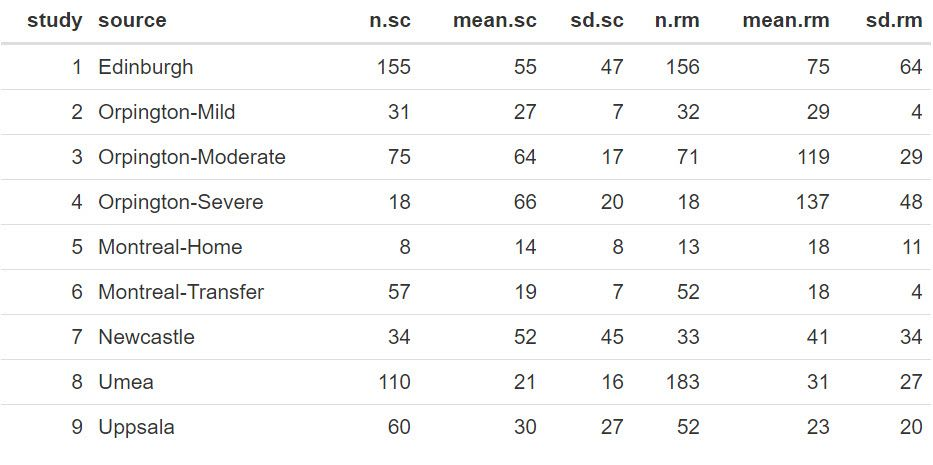### Statistical analyses

We will use the statistical package R to read and manipulate the data, and then the library ASReml-R (Butler et al. 2017) to fit the models.
First, we read the data in R and make some additional calculations, as shown in the code below:

 STROKE <- read.table("STROKE.TXT", header=TRUE)
 STROKE$diff <- STROKE$mean.sc - STROKE$mean.rm STROKE$Vdiff <- (STROKE$sd.sc^2/STROKE$n.sc) + (STROKE$sd.rm^2/STROKE$n.rm) STROKE$WT <- 1/(STROKE$Vdiff)

The new column diff contains the difference between treatment means (as reported from each study). We have estimated the variance of this mean difference, Vdiff, by taking from each treatment its individual MSE (mean square error) and dividing it by the sample size, and then summing the terms of both treatments. This estimate assumes, that for a given study, the samples from both treatments are independent, and for this reason we did not include a covariance. Finally, we have calculated a weight (WT) for each study as the inverse of the variance of the mean difference (i.e., 1/Vdiff).

We can take another look at this data with these extra columns: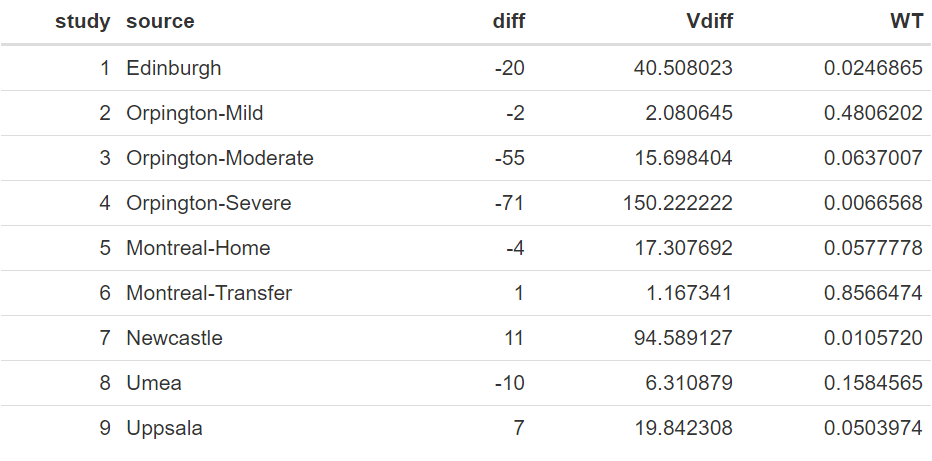The above table shows a wide range of values between the studies in the mean difference of length of stay between the two treatments, ranging from as low as −71.0 to 11.0, with a raw average of −15.9. Also, the variances of these differences vary considerably, which is also reflected in their weights.

The code to fit the fixed-effect linear model using ASReml-R is shown below:

 library(asreml) meta_f<-asreml(fixed=diff~1,                weights=WT,                family=asr_gaussian(dispersion=1),                data=STROKE)

In the above model, our response variable is diff, and the weights are indicated by the variate WT. As the precisions are contained within the weights the command family is required to fix the residual error (MSE) to exactly 1.0, hence, it will not be estimated.

The model generates output that can be used for inference. We will start by exploring our target parameter, i.e. θ, by looking at the estimated fixed effect mean and its standard error. This is done with the code:

 meta_effect <- summary(meta_f, coef=TRUE)$coef.fixed Resulting in the output: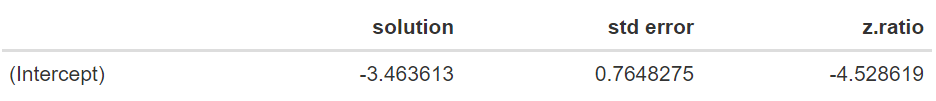The estimate of θ is equal to −3.464 days, with a standard error of 0.765. An approximate 95% confidence interval can be obtained by using a z-value of 1.96. The resulting approximate 95% confidence interval [−4.963;−1.965] does not contain zero. The significance of this value can be obtained by looking at the approximated ANOVA table using the command:  wald.asreml(meta_f) Note that this is approximated as, given that weights are considered to be known, the degrees of freedom are assumed to be infinite; hence, this will be a liberal estimate.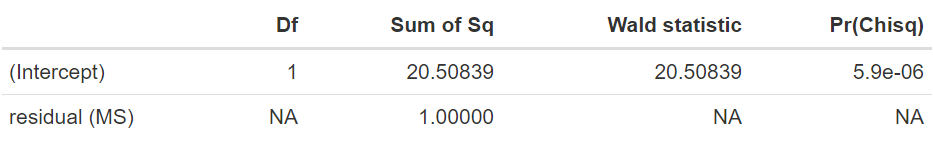The results from this ANOVA table indicate a high significance of this parameter (θ) with an approximated p-value of < 0.0001. Therefore, in summary, this fixed effect model analysis indicates a strong effect of the specialised care resulting in a reduction of approximately 3.5 days in hospitalisation. However, as indicated earlier, a random-effects model might seem more reasonable given the inherent differences in the studies under consideration. Here, we extend the model to include the random effect of study. In order to do this, first we need to ensure that this is treated as a factor in the model by running the code:  STROKE$study <- as.factor(STROKE$study)_f) The LMM to be fitted using ASReml-R is:  meta_r<-asreml(fixed=diff~1,  random=~study,  weights=WT,  family=asr_gaussian(dispersion=1),  data=STROKE) Note in this example the only difference from the previous code is the inclusion of the line random=~study. This includes the factor study as a random effect. An important result from running are the variance component estimates. These are obtained with the command:  summary(meta_r)$varcomp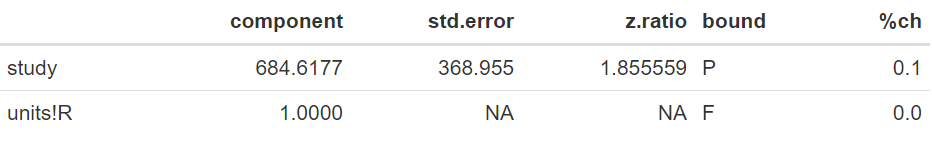In this example, the variance associated with the differences in the target parameter (θ) between the studies is 684.62. When expressed as a standard deviation, this corresponds to 26.16 days. Note that this variation is large in relation to the scale of the data, reflecting large differences between the random sample of studies considered in the meta-analysis.

We can output the fixed and random effects using the following commands:

 meta_effect <- summary(meta_r, coef=TRUE)$coef.fixed BLUP <- summary(meta_r, coef=TRUE)$coef.random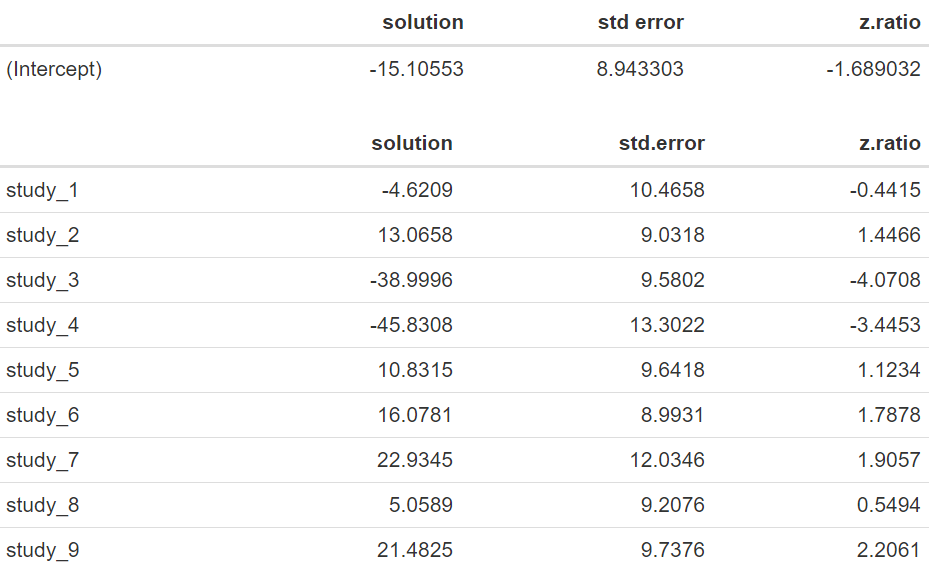Note that now that our estimated mean difference corresponds to −15.106 days with an standard error of 8.943, and that the approximate 95% confidence interval [−32.634;2.423] now contains zero. An approximated ANOVA based on the following code:

 wald.asreml(meta_r)

results in the output: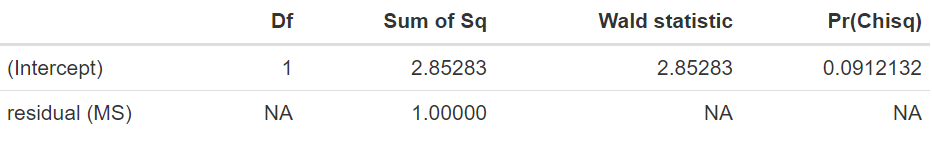We have a p-value of 0.0912, indicating that there is no significant difference in length of stay between the treatments evaluated. Note that the estimates of the random effects of study, also known as BLUPs (best linear unbiased predictions) are large, ranging from −45.8 to 22.9, and widely variable. The lack of significance in the random-effects model, when there is a difference of −15.11 days, is mostly due to the large variability of 684.62 found between the different studies, resulting in a substantial standard error for the estimated mean difference.

In the following graph we can observe the 95% confidence intervals for each of the nine studies together with the final parameter estimated under the Random-effects Model. Some of these confidence intervals contain the value zero, including the one for the random-effects model. However, it can be observed that the confidence interval from the random-effects model is an adequate summarization of the nine studies, representing a compromising confidence interval.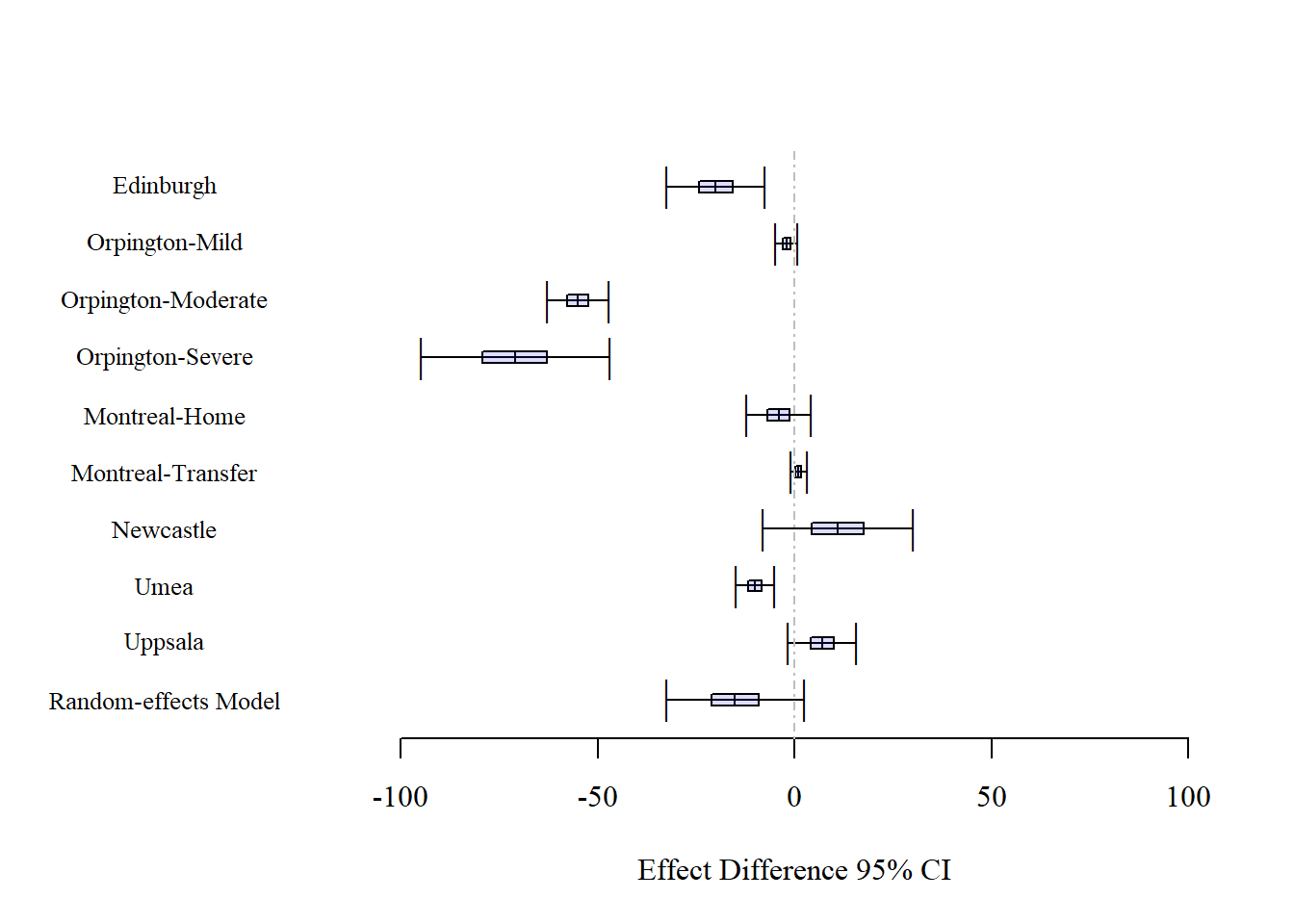An important aspect to consider is the difference in results between the fixed-effect and the random-effects model that are associated, as indicated earlier, with different inferential approaches. One way to understand this is by considering what will happen if a new random study is included. Because we have a large variability in the study effects (as denoted by ), we expect this new study to have a difference between treatments that is randomly within this wide range. This, in turn, is expressed by the large standard error of the fixed effect θ, and by its large 95% confidence interval that will ensure that for ‘any’ observation we cover the parameter estimate 95% of the time. Therefore, as shown by the data, it seems more reasonable to consider the random-effects model than the fixed-effect model as it is an inferential approach that deals with several sources of variation.

### Summary

In summary, we have used the random-effects model to perform meta-analysis on a medical research question of treatment differences by combining nine independent studies. Under this approach we assumed that all studies describe the same effect but we allowed for the model to express different effect sizes through the inclusion of a random effect that will vary from study to study. The main aim of this analysis was not to explain why these differences occur, here, our aim was to incorporate a measure of this uncertainty on the estimation of the final effect of treatment differences.

There are several extensions to meta-analysis with different types of responses and effects. Some of the relevant literature recommended to the interested reader are van Houwelingen et al. (2002) and Vesterinen et al. (2014). Also, a clear presentation with further details of the differences between fixed-effect and random-effects models is presented by Borenstein et al. (2010).

Dataset: STROKE.txt
R code: STROKE_METAA.R

### References

Borenstein, M; Hedges, LV; Higgins, JPT; Rothstein, HR. 2010. A basic introduction to fixed-effect and random-effects models for meta-analysis. Research Synthesis Methods 1: 97-111.

Butler, DG; Cullis, BR; Gilmour, AR; Gogel, BG; Thompson, R. 2017. ASReml-R Reference Manual Version 4. VSNi International Ltd, Hemel Hempstead, HP2 14TP, UK.

Normand, ST. 1999. Meta-analysis: Formulating, evaluating, combining, and reporting. Statistics in Medicine 18: 321-359.

van Houwelingen, HC; Arends, LR; Stignen, T. 2002. Advanced methods in meta-analysis: multivariate approach and meta-regression. Statistics in Medicine 21: 589-624.

Vesterinen, HM; Sena, ES; Egan, KJ; Hirst, TC; Churolov, L; Currie, GL; Antonic, A; Howells, DW; Macleod, MR. 2014. Meta-analysis of data from animal studies: a practical guide. Journal of Neuroscience Methods 221: 92-102.